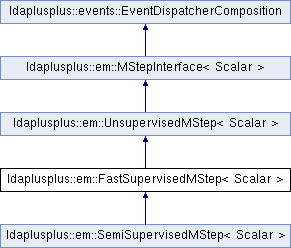LDA++
ldaplusplus::em::FastSupervisedMStep< Scalar > Class Template Reference

#include <FastSupervisedMStep.hpp>

Inheritance diagram for ldaplusplus::em::FastSupervisedMStep< Scalar >:## Public Member Functions

FastSupervisedMStep (size_t m_step_iterations=10, Scalar m_step_tolerance=1e-2, Scalar regularization_penalty=1e-2)

virtual void m_step (std::shared_ptr< parameters::Parameters > parameters) override

virtual void doc_m_step (const std::shared_ptr< corpus::Document > doc, const std::shared_ptr< parameters::Parameters > v_parameters, std::shared_ptr< parameters::Parameters > m_parameters) overridePublic Member Functions inherited from ldaplusplus::events::EventDispatcherComposition
std::shared_ptr< EventDispatcherInterfaceget_event_dispatcher ()

void set_event_dispatcher (std::shared_ptr< EventDispatcherInterface > dispatcher)

## Detailed Description

### template<typename Scalar> class ldaplusplus::em::FastSupervisedMStep< Scalar >

Implement the M step for the fsLDA.

Similarly to the UnsupervisedMStep the purpose is to maximize the lower bound of the log likelihood $$\mathcal{L}$$. The same notation as in UnsupervisedMStep is used.

$\log p(w, y \mid \alpha, \beta, \eta) \geq \mathcal{L}(\gamma, \phi \mid \alpha, \beta, \eta) = \mathbb{E}_q[\log p(\theta \mid \alpha)] + \mathbb{E}_q[\log p(z \mid \theta)] + \mathbb{E}_q[\log p(w \mid z, \beta)] + H(q) + \mathbb{E}_q[\log p(y \mid z, \eta)]$

We observe that with respect to the parameter $$\beta$$ nothing changes thus FastSupervisedMStep extends UnsupervisedMStep to delegate part of the maximization to it. Decoration or another type of composition may be a more appropriate form of code reuse in this case.

To maximize with respect to $$\eta$$ we use the following equation which amounts to simple logistic regression. The reasons for this approximation are explained in our ACM MM '16 paper (to be linked when published).

$\mathcal{L}_{\eta} = \sum_d^D \eta_{y_d}^T \left(\frac{1}{N} \sum_n^{N_d} \phi_{dn}\right) - \sum_d^D \log \sum_{\hat y}^C \text{exp}\left( \eta_{\hat y}^T \left(\frac{1}{N} \sum_n^{N_d} \phi_{dn}\right) \right)$

This implementation maximizes the above equation using batch gradient descent with ArmijoLineSearch.

## Constructor & Destructor Documentation

template<typename Scalar >
 ldaplusplus::em::FastSupervisedMStep< Scalar >::FastSupervisedMStep ( size_t m_step_iterations = 10, Scalar m_step_tolerance = 1e-2, Scalar regularization_penalty = 1e-2 )
inline
Parameters
 m_step_iterations The maximum number of gradient descent iterations m_step_tolerance The minimum relative improvement between consecutive gradient descent iterations regularization_penalty The L2 penalty for logistic regression

## Member Function Documentation

template<typename Scalar >
 void ldaplusplus::em::FastSupervisedMStep< Scalar >::doc_m_step ( const std::shared_ptr< corpus::Document > doc, const std::shared_ptr< parameters::Parameters > v_parameters, std::shared_ptr< parameters::Parameters > m_parameters )
overridevirtual

Delegate the collection of some sufficient statistics to UnsupervisedMStep and keep in memory $$\mathbb{E}_q[\bar z_d] = \frac{1}{N} \sum_n^{N_d} \phi_{dn}$$ for use in m_step().

Parameters
 doc A single document v_parameters The variational parameters used in m-step in order to maximize model parameters m_parameters Model parameters, used as output in case of online methods

Reimplemented from ldaplusplus::em::UnsupervisedMStep< Scalar >.

Reimplemented in ldaplusplus::em::SemiSupervisedMStep< Scalar >.

template<typename Scalar >
 void ldaplusplus::em::FastSupervisedMStep< Scalar >::m_step ( std::shared_ptr< parameters::Parameters > parameters )
overridevirtual

Maximize the ELBO w.r.t. to $$\beta$$ and $$\eta$$.

Delegate the maximization regarding $$\beta$$ to UnsupervisedMStep and maximize $$\mathcal{L}_{\eta}$$ using gradient descent.

Parameters
 parameters Model parameters (changed by this method)

Reimplemented from ldaplusplus::em::UnsupervisedMStep< Scalar >.

The documentation for this class was generated from the following files: